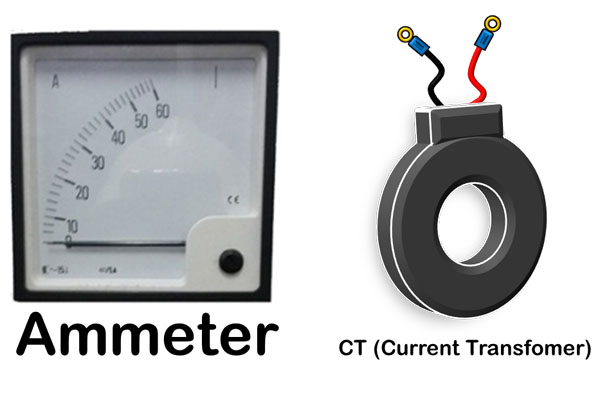# Working Principles of AMMETER and CT (Current Transformer)

Get to know the working principle of Ammeters and CT (Current Transformer), and the calculations
Ammeters is a device to measure electric current in a closed circuit, and CT is a device used to receive magnetic induction from an conductor when electric current is flowed, and convert it into a smaller electric current value according to the comparison of the CT ratio .

Ampere is a unit to express the magnitude of an electric current, and the amount of electric current flowing in an electrical circuit can be measured using an electric current measuring, commonly referred to as Ammeters.

In an Electric Panel, there are various components, be it the main component, Control (Control), and measuring instrument, and the Ammeters is one of instrument measuring instruments that are often used in an electric panel.

## Working Principles of AMMETER and CT (Current Transformer), and how to calculatedAmmeter and Current Transformer
Ammeters
Ammeters is used to measure how big electric current (in Ampere units) flows in a closed electrical circuit, and usually it is installed and connected in series on an electrical circuit.

Working principle of Ammeters and CTDiagram of Current measurement
Ammeters works based on magnetic induction force generated on the coil inside, when an electric current flows and passes through the coil it will produce magnetic induction, then the magnetic force produced will be used to move the Measuring Needle according to the amount of the current.

Installation of Ammeters for measuring small Current is connected in series circuit, but if the Current measured has a big value, usually Ammeters will require with CT (Current Transformer) to convert the measurement results to Flow large electricity becomes smaller, then converted back to the actual value of electric current.

Given the greater the electric current to be measured, the bigger the Ammeters needed as a measuring instrument, then CT (Current Transformer) is used to measure the value of a large electric current, but it is converted to smaller before flowing to the Ammeters.

CT (Current Transformer)
CT (Current Transformer) is one type of transformer that has 2 types of coils in it, namely Primary coils in the form of plate plates which are layered to receive magnetic induction from actual electric current, and Secondary coils to receive magnetic induction generated by the Primer and convert it to a smaller value of Electric Current (Amperage) according to the ratio (Comparison) of the coils on the CT.

Calculation of CT ratio and electric current measured
Ammeters that uses CT (Current Transformer) to measure electric current, there is a Ratio value (comparison), and the value of the CT and Ammeters ratios that are widely used are ... / 5A.

What is the ratio ... / 5A?

The ratio of ... / 5A to a CT (Current Transformer) and Ammeters has the following meanings:
• ... is the maximum value of current measurement capability in an Ammeters or CT.
• / 5A is the maximum current value received by Ammeters or the CT output is 5Ampre, when the maximum current value is measured.

Example:
In an electric panel, use an Ammeters with a value of 100 / 5A, and the installed CT also has a value of 100 / 5A.

Then when the electric current in the electrical panel circuit flows by 100 Ampere, CT will capture the induction of the electrical circuit by 100 Amperes in the Primary Coils, then the Secondary Coils decreases the Electric current to 5A, and sends an electric current of 5 Ammeters, and then the Ammeters will convert the 5 Ampere electric current again to 100Ampere according to the actual measurement results.

100 / 5A means "Every measured electric current is 100Amperes, then it is converted to 5Amperes"

Then, when the current is 80 Ampere, CT will change the value of 80 Amperes to: 4 amperes, with calculations, as follows:

80Ampere : (100/5)

80 Amperes : 20 = 4 Amperes.

If the actual current value is 80Ampere, then CT 100/5 will convert it to 4 Ampere, and then the electric current value is flowed to the Ammeters to be converted back to be shown to be the actual current value of 80 Ampere.

Then what is the calculation for CT 1000/5A?

CT with a ratio of 1000/5A, this means that the conversion value is 1000 divided by 5 equals 200, So every electric current that flows by 200 Ampere, then CT will convert it to a current value of 1 A, as well as if the electric current that flows by 400 Ampere CT will change it to 2 Ampere.

The calculation formula:
Current divided by ratio (... / 5A)
If the CT used is written 1000/5, then the conversion value is 200 (Every measured electric current is 200Ampere, then CT will produce an electric current of 1Ampere), If the CT used is written 4000/5, then the conversion value is 800 (Every electric current measured is 800Ampere, then CT will produce an electric current of 1Ampere)

Likewise with the CT working principle, which is attached to a 3 phase KWH meter, to calculate electrical power consumption (KWH), the measurement results indicated by KWH meters must be multiplied again by the CT ratio used.

My Electrical Diary